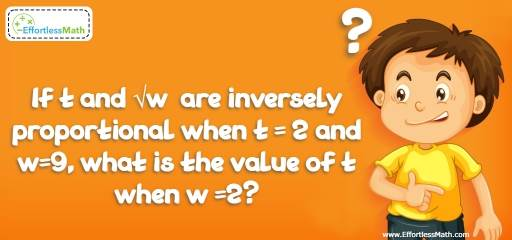# Number Properties Puzzle – Challenge 13

If you are looking for maths puzzles with answers, you are in the right place. The solution can be very useful when trying to solve a hard critical thinking challenge.## Challenge:

If t and $$\sqrt{w}$$ are inversely proportional when t = 2 and w=9, what is the value of t when w =2?

### The Absolute Best Book to challenge your Smart Student!

The correct answer is $$3\sqrt{2}$$.

t and $$\sqrt{w}$$ are inversely proportional when t = 2 and w=9,
Then, $$t × \sqrt{w} = 2 × \sqrt{9} = 2 × 3 = 6$$
w =2, then,
$$t × \sqrt{w} = 6 → t × \sqrt{2} = 6 → t = \frac{6}{\sqrt{2}} × \frac{\sqrt{2}}{\sqrt{2}} = \frac{6\sqrt{2}}{2} = 3\sqrt{2}$$

### What people say about "Number Properties Puzzle – Challenge 13 - Effortless Math: We Help Students Learn to LOVE Mathematics"?

No one replied yet.

X
30% OFF

Limited time only!

Save Over 30%

SAVE $5 It was$16.99 now it is \$11.99